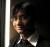# Data values are going inside the plot column

## Recommended Posts

Hi All, I am using MSColumn chart v3.0. and i am facing the problem that the data values to be displayed on the columns are going inside the columns when there is no space for these data values. Please let me know if i can arrange some space for these data values.

Thanks

Mahesh

##### Share on other sites

Please have a look into the xml. and suggest the solution

chartXML.txt

##### Share on other sites

Hi,

Could you please try using YAxisMaxValue='15' & YAxisMinValue='-15' , using it'll create a gap from top & bottom, which leaves some space to the dataPlot value.

The values of these attributes should be maximum value in the dataset + 4 for negative value as well as for positive value.

<chart YAxisMaxValue='15' YAxisMinValue='-15'  adjustDiv='0' setAdaptiveYMin='1' zeroPlaneAlpha='30' showShadow='1' showValues='1' animation= '1' numdivlines='0' showDivLineValue='0' showToolTip='1' toolTipBgColor='F8F8F8' showPlotBorder='1' plotBorderColor='FDFDFD' plotFillAngle='360' plotFillRatio='100' plotGradientColor='F8F8F9' showLimits='0' showLegend='0' canvasBorderColor='FFFFFF' canvasBorderThickness='0' bgColor='FFFFFF' showBorder='0' chartLeftMargin='0' chartRightMargin='0' chartBottomMargin='0' chartTopMargin='5' valuePadding='0' baseFont='Arial' baseFontSize='11' baseFontColor='999999' baseFont='Arial' outCnvbaseFontSize='11' outCnvBaseFontColor='000000'>

<categories>

<category label='jan' showLabel='1'/>

<category label='feb' showLabel='1'/>

<category label='mar' showLabel='1'/>

<category label='apr' showLabel='1'/>

<category label='may' showLabel='1'/>

<category label='jun' showLabel='1'/>

<category label='sss' showLabel='1'/>

<category label='ssw' showLabel='1'/>

<category label='ssw' showLabel='1'/>

<category label='ssw' showLabel='1'/>

<category label='ssw' showLabel='1'/>

<category label='ssw' showLabel='1'/>

</categories>

<dataset  seriesName='' color='FF0000' >

<set value='-4'/>

<set value='-5'/>

<set value='-5'/>

<set value='-1'/>

<set value='-6'/>

<set value='3'/>

<set value='7'/>

<set value='11'/>

<set value='9'/>

<set value='6'/>

<set value='2'/>

<set value='-1'/>

</dataset>

<dataset  seriesName='' color='0033CC' >

<set value='-4'/>

<set value='-5'/>

<set value='-5'/>

<set value='-1'/>

<set value='-6'/>

<set value='3'/>

<set value='7'/>

<set value='11'/>

<set value='9'/>

<set value='6'/>

<set value='2'/>

<set value='-1'/>

</dataset>

</chart>